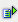The function sumsq (range) calculates the sum of the squares of the values within a range.

Syntax: =sumsq(range)Example

Cells A1 to A10: any numbers

Cell A11 = sumsq(A1:A10)

 R / C A B 1 1 1 2 2 4 3 3 9 4 4 16 5 5 25 6 6 36 7 7 49 8 8 56 9 9 81 10 10 100 11 385 385

Supervision:

Cell B1 to B10: Squares of A1 to A10

Cell B11 = sum(B1:B10)